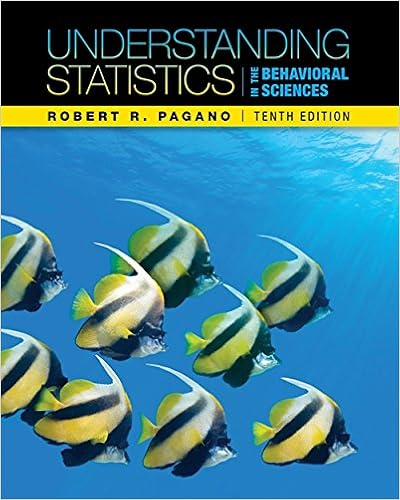# ch6 stat - The Practice of Statistics Yates Moore Starnes...

• Homework Help
• 3

This preview shows page 1 - 3 out of 3 pages.

##### We have textbook solutions for you!
The document you are viewing contains questions related to this textbook.The document you are viewing contains questions related to this textbook.
Chapter 8 / Exercise 8
Understanding Statistics in the Behavioral Sciences
PaganoExpert Verified
The Practice of Statistics- Yates, Moore, & StarnesChapter 6: ProbabilityKey Vocabulary:trialrandomprobabilityindependencerandom phenomenonsample spaceS = {H, T}tree diagramreplacementeventP(A)Complement ACdisjointVenn Diagramunion (or)intersection (and)joint eventjoint probabilityconditional probability6.1Randomness (pp.330-335)1.In statistics, what is meant by the term random2.In statistics, what is meant by probability3.What is probability theory4.In statistics, what is meant by an independenttrial????Chapter 6: Probability: The Study of Randomness
##### We have textbook solutions for you!
The document you are viewing contains questions related to this textbook.The document you are viewing contains questions related to this textbook.
Chapter 8 / Exercise 8
Understanding Statistics in the Behavioral Sciences
PaganoExpert Verified
The Practice of Statistics- Yates, Moore, & Starnes6.2Probability Models (pp.335-359)1.In statistics, what is a sample space2.In statistics, what is an event??
•••